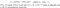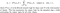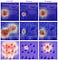A Generic Visualization Approach for Convolutional Neural Networks

This paper  proposes a tool, L2-CAF, to visualize attention in convolutional neural networks. L2-CAF is a generic visualization tool that can do everything CAM  and Grad-CAM  can do, but the opposite is not true.

Given a pre-trained CNN, an input x generates an output NT(x) — this is the solid green path in the next Figure. For the same input x, if the last convolutional layer’s output is multiplied by a constrained attention filter f, the network generates another output FT(x,f) — this is the dashed orange path. The filter f is randomly initialized then optimized using gradient descent. The optimization objective function L is to minimize the difference between NT(x) and FT(x,f). The filter f is constrained to identify and block irrelevant/background features contributing nothing to NT(x).

Formally, L2-CAF formulates attention visualization as a constrained optimization problem with the following minimization objective.L2-CAF mathematical formula. Using gradient descent, optimize the filter f that minimizes the constrained objective L.

This formula explains the name L2-CAF, which is short for “unit L2-Norm Constrained Attention Filter”. L2-CAF is a generic visualization tool because it makes no assumptions about the network architecture. The input x can be a regular image or a pre-extracted convolutional feature. The network output can be logits trained with softmax or a feature embedding trained with a ranking loss. Furthermore, this approach neither changes the original network weights nor requires fine-tuning. Thus, network performance remains intact. The visualization filter is applied only when an attention map is required. Thus, it poses no computational overhead during inference. L2-CAF visualizes the attention of the last convolutional layer of GoogLeNet within 0.3 seconds.

The previous formula is class-oblivious because it generates a single attention map per input x. If x has multiple objects, it is difficult to attend to distinct objects. L2-CAF has another class-specific version to generate different attention maps for different objects in the input. The next equation shows the L2-CAF class-specific formula.The class-specific L2-CAF formula. The constraint objective L maximizes the logit for a particular class while minimizing the logits for all other classes.

The next figure highlights the difference between the class-oblivious and class-specific versions. The class-oblivious attention map (left) highlights both the dog and the butterfly, while the class-specific generates both dog (middle) and butterfly (right) specific attention maps.

The class-oblivious and class-specific L2-CAF versions explain why L2-CAF is a generic visualization approach. CAM  and Grad-CAM  assume a classification network with logits output while L2-CAF does not. L2-CAF works for both classification and feature embedding networks. CAM  requires a global average pooling (GAP) layer inside the network, L2-CAF does not make any assumption about the network architecture. Thus, L2-CAF can do everything CAM and Grad-CAM can do, but the opposite is not true.

The constrained attention filter (CAF) requires constraint to avoid the trivial solution in which f degenerates into an all-one filter ({1}^{w\times h}). CAF supports various constraints such as softmax and Gaussian. Yet, the intuition behind L2-CAF is that an ideal attention map can be regarded as a filter that approximates NT(x) by blocking irrelevant features. Accordingly, we seek a filter f that spatially prioritizes convolutional features and flexibly captures irregular (e.g., discontinuous) shapes or multiple different agents in a frame. The L2-Norm, a simple multi-mode differentiable filter, satisfies these requirements. On account of irrelevant features, the ||f||_2=1 constraint assigns higher weights to relevant features. The next figure highlights the benefit of a multi-mode constraint when the input x has more than a single object.Qualitative evaluation for alternative attention constraints. A softmax constraint offers a sparse attention map, while a Gaussian constraint assumes a single-mode (object). The L2-Norm constraint supports multi-mode (objects).

L2-CAF leverages gradient descent to minimize the constraint objective. Thus, L2-CAF is slower than Grad-CAM but this iterative nature has a cool benefit. It is possible to see the filter converging to its solution. The next video shows randomly initialized filters converging on images with multiple objects.